Need Help?

Subscribe to Statics

###### \${selected_topic_name}
• Notes
• Comments & Questions

Determine the horizontal and vertical components of reaction at pins  $A$  and  $C$  of the two-member frame.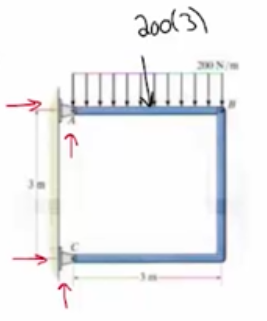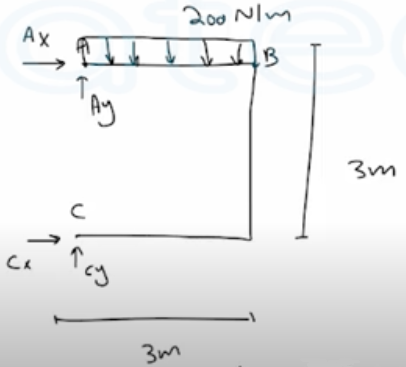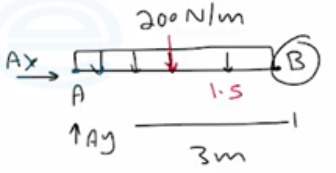$\sum M_{B}=0$

$200(3)(1.5)-A _y(3)=0$

$∴ A _y=300 N$

$+\uparrow \sum F_{y}=0$

$A_ y+(y-200(3))=0$

$C _y=600-300=300 N$

$\sum M_{A}=0$

$C_{x}(3)-(200)(3)(1.5)=0$

$∴ C_x=300 N$

$\stackrel{+}{\longrightarrow} \sum F_{x}=0$

$c_ x+A_ x=0 \longrightarrow\quad A_x=300N\leftarrow$

Two beams are connected together by the short  link  $B C$ . Determine the components of reaction at the fixed  support  $A$  and at pin $D$.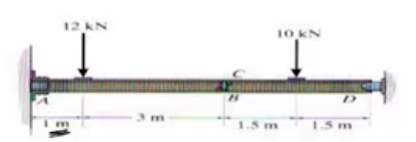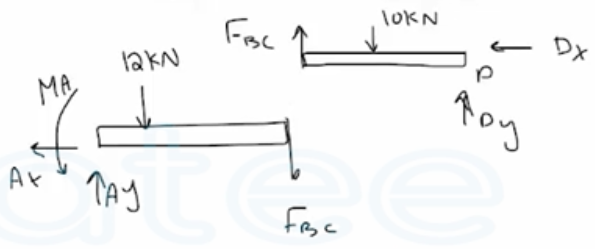BD

$\sum M_{p}=0$
$10(1.5)-F_{B C}(3)=0$

$∴ F_{B C}=5 K N$

$\stackrel{+}\longrightarrow\sum F_{x}=0 \quad \longrightarrow D_{x}=0$

$+\uparrow \sum F_ y=0 \quad \longrightarrow \quad D_y-10+5=0$

$D_ y=5 k N$

AC

$\stackrel{+}\longrightarrow\sum F_{x}=0 \quad \longrightarrow A_{x}=0$

$+\uparrow \sum F_ y=0 \quad \longrightarrow \quad A_y-12-5=0 \quad \longrightarrow A_y=17kN$

$\sum M_ A=0\quad\longrightarrow\quad M_A-(12)(1)-5(4)=0 \ \longrightarrow M_A=32kN.m$

No comments yet